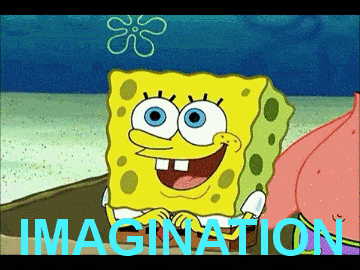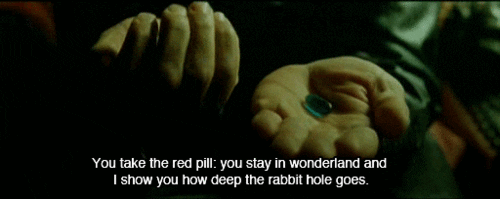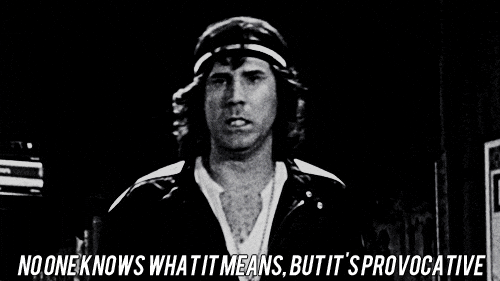## How to prove there is no Universe

Years ago, when I first read Paul Halmos’ seminal Naive Set Theory, I was blown away by how easy it was to prove that there is no universe. In fact, not even three sections in, he drops this italicized bombshell:

$$nothing\text{ }contains\text{ }everything$$

or, “more spectacularly,” he continues

$$there\text{ }is\text{ }no\text{ }universe$$

Luckily, we only need two axioms to prove this:

• The Axiom of Extension
• The Axiom of Specification

In this post, I’ll give an accessible and (hopefully) fun introduction to this interesting property of modern foundational mathematical systems. An axiom, in case you’re wondering, is a truth a logical system takes to be self-evident. Note that an axiom doesn’t necessarily have to be uncontroversial (in fact, many are!), but it has to be regarded as true for us to do anything fun inside a given system. Sometimes, axioms are even used as a narrative technique; here’s Thomas Jefferson:

We hold these truths to be self-evident: that all men are created equal; that they are endowed by their Creator with certain unalienable rights; that among these are life, liberty, and the pursuit of happiness.

Everything that follows in the Declaration of Independence, follows from these claims. But let’s get back to Halmos. We’ll be using standard set notation, emojis, and our imagination.### The Axiom of Extension

This axiom is pretty easy to grasp. The technical definition goes something like this:

Two sets are equal if and only if they have the same elements

Consider the set of the most used emojis on my iPhone:

$$\{?, ?, ?, ?\}$$

Trivially, then:

$$\{?, ?, ?, ?\} = \{?, ?, ?, ?\}$$

And also:

$$\begin{array}{l} A=\{?,?,?,?\}\\ B=\{?,?,?,?\}\\ A=B \end{array}$$

Before we get into the Axiom of Specification, it would be prudent to make a clear distinction between a set of a thing and the thing itself. In other words, these two are not the same:

$$\{?\}\neq ?$$

### The Axiom of Specification

The next axiom, of Specification, is a bit trickier; the definition goes like so:

If $$A$$ is a set and $$S(x)$$ is a logical condition, then there is a set $$B$$ whose elements are exactly those $$x \in A$$ such that $$S(x)$$ is true.

We read$$\text{ } \in \text{ }$$as “is an element of” or “in” and$$\text{ } \notin \text{ }$$as “is not an element of” or “not in.” Suppose we have a farmer named Bob. Let the set of Bob’s farm animals be $$F$$ and let $$S(x)$$ be the condition “$$x$$ has feathers”. The set of all animals in $$F$$ that have feathers is defined like so:

$$P=\{\,x\in F \mid x \text{ has feathers}\,\}$$

This way of writing sets is called set-builder notation. We can read the above as “Get me all the feathery things inside $$F$$,” or, more formally, “$$x$$ such that $$x$$ is an element of $$F$$ and $$x$$ has feathers.” We call this new set $$P$$ (for poultry). If Bob has a chicken and a duck, then we might end up with this:

$$P=\{?,?\}$$

If Bob only has a turkey, we’ll end up with this:

$$P=\{?\}$$

Okay, now that we know the rules, let’s do some cool stuff.

### The Universe is a Lie

So we know that $$S(x)$$ is just a condition; any condition, in fact. Above, this condition was “$$x$$ has feathers.” But what happens if we make the condition “$$x$$ is not in $$x$$“? Or, more succinctly:

$$x \notin x$$

Don’t worry about what exactly “$$x$$ not in $$x$$” means in the real world; simply put, $$x$$ seems to be a set and the condition is that it doesn’t contain itself. So we have our condition, even though it may not seem to make much sense. Let’s build a new set!

$$B=\{\,x\in A \mid x \notin x\,\}$$

That wasn’t too hard. Now let’s think about what $$B$$ will contain. I have no idea what crazy stuff we’ll find, so I’ll just use a $$?$$ as a placeholder for any thing inside $$B$$. We know exactly two properties of things in $$B$$:

• $$? \in A$$
• $$? \notin ?$$

In other words, for $$?$$ to be in $$B$$, it must also be in $$A$$, and it also can’t contain itself. Written more rigorously, we have:

$$\text{?}\in B \iff (\text{?}\in A\text{ and }\text{?}\notin\text{?})$$

Where $$\iff$$ means “if and only if”. Okay, so far, so good. Nothing really interesting yet and it seems like we’re going down a rabbit hole for no good reason, but we need just one more leap: is it possible that $$B \in A$$?And here starts the proof. First, let’s just assume that $$B \in A$$. Next, we’re going to use the vacuously true statement “P or not P” to prove a contradiction. Even in regular day-to-day conversations, this logical tool seems intuitive: “either you got an A or you didn’t get an A”; “either you went to the store or didn’t go to the store”; “you fixed your car or you didn’t fix your car”; all these statements are tautologies, in other words “uh, duh.” We’re going to apply that to our proof; so, we’re going to say: given our assumption, either “$$B$$ is in $$B$$” or “$$B$$ is not in $$B$$.” These statements should be met with a logical “duh,” but let’s see what happens.

Looking at the $$B \in B$$ case first, let’s remember our assumption ($$B \in A$$) and go from there. To get what we’re looking for, all we need to do is use the biconditional above and fill in our assumptions:

$$B\in B \iff (B \in A\text{ and }\text{?}\notin\text{?})$$

So it seems that all $$?$$ are being uniformly replaced with $$B$$. That makes sense; $$?$$ was just a placeholder. Let’s finish up the substitution:

$$B\in B \iff (B \in A\text{ and }B \notin B)$$

Uh oh, looks like we’re getting a contradiction: $$B\in B$$ on the left side and $$B \notin B$$ on the right side. That can’t be right. It’s crazy to say that you washed your car and you didn’t wash your car. Okay, so that’s a dead end, but what about the $$B \notin B$$ case? Again, we recall our original assumption ($$B \in A$$) and substitute:

$$\text{?}\in B \iff (B \in A\text{ and }B \notin B)$$

$$B\in B \iff (B \in A\text{ and }B \notin B)$$

The same contradiction. Darn. Okay, so because both cases ended up in contradictions, it looks like our original assumption — that $$B \in A$$ — was false. So, we officially proved the opposite: that $$B \notin A$$. But what does this mean?I snuck this by you, dear reader, but I never actually defined $$A$$. $$A$$ is as big, or as small, as you want it to be. Let’s say we want $$A$$ to be the universe: a humongous set that contains all other things; we can even throw in all the things that we can’t even think of for good measure. This set has everything in it. But here’s the kicker: we just proved that there’s a thing out there $$B$$ that’s not in this universe $$A$$. Well, a universe which doesn’t contain all things is hardly a universe, so we end up with the awesomely stunning conclusion that there is no universe!

### It’s my Universe and I need it now!

(What follows is a bit more technical, but interesting nonetheless.)

If you’re a bit troubled by this, you’re not the only one. People liked the idea of a universal set (in fact, many still do). Prior to the early 20th century, the universal set was taken for granted in logic and mathematics.

You probably realized that there’s something funky going on with the Axiom of Specification — it seems to embed the impossibility of a universal set in its definition. In fact, that’s precisely it’s purpose. This axiom is sometimes called the Axiom of Restricted Comprehension, and we’ll see why in a moment. Prior to Restricted Comprehension, we had the much more powerful Unrestricted Comprehension: a Frankenstein of Gottlob Frege’s creation. According to Frege, a set could be defined arbitrarily given any condition $$\phi(x)$$:

$$\{\,x \mid \phi(x)\,\}$$

The set of all sets, also known as the universal set, can be defined like so:

$$\{\,x \mid x=x\,\}$$

But Bertrand Russel discovered that if we set the condition to $$x \notin x$$, we end up with the following:

\begin{array}{lll}
1. & A=\{\,x\mid x\notin x\,\} & \text{Define A}\\
2. & A\in A\Rightarrow A\notin A & \text{From (1)}\\
3. & A\notin A\Rightarrow A\in A & \text{From (1)}\\
4. & A\in A\iff A\notin A & \text{From (2) and (3)}\\
This is known as Russell’s Paradox. And that’s why set creation is restricted in foundational mathematics and in most other modern logics; in metalogic, for example, we must define the domain of discourse, e.g., let the model for a language $$\mathfrak{L}$$ be the triple $$\mathfrak{M}=(\boldsymbol{D},v,\chi)$$ where:
1. $$\boldsymbol{D}$$ is a nonempty set (the domain or universe of $$\mathfrak{M}$$)
2. $$v$$ is the valuation function
3. $$\chi$$ is the constant assignment of $$\mathfrak{M}$$